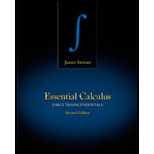# The inequality 1 3 &lt; ln 1.5 &lt; 5 12 by comparing areas.### Essential Calculus: Early Transcen...

2nd Edition
James Stewart
Publisher: Cengage Learning
ISBN: 9781133112280### Essential Calculus: Early Transcen...

2nd Edition
James Stewart
Publisher: Cengage Learning
ISBN: 9781133112280

#### Solutions

Chapter C, Problem 1E

(a)

To determine

## To show: The inequality 13<ln1.5<512 by comparing areas.

Expert Solution

### Explanation of Solution

Definition used:

The natural logarithmic function is the function defined by lnx=1x1tdt,x>0.

If x>1, then lnx can be interpreted geometrically as the area under the hyperbola y=1t from t = 1 to t = x.

Calculation:

The given inequality is 13<ln1.5<512.

Here, x>1.

By using the above mentioned definition, ln1.5 can be interpreted geometrically as the area under the hyperbola y=1t from t = 1 to t = 1.5. (1,1), (1,23)(32,23)

Use online graphing calculator, graph of the curve y=1t as shown in Figure 1.From Figure 1, it is observed that the area is larger than the area of rectangle BCDE and smaller than the area of trapezoid ABCD.

The area of the rectangle BCDE is calculated as follows.

Area of BCDE=lw=(321)(230)=(322)23=12(23)=13

The area of the trapezoid ABCD is calculated as follows.

Area of ABCD=12(a+b)h=12(230+1)(1.51)=12(2+33)(0.5)=12(53)12=512

It is known that the value of ln1.5 is 0.40546.

The decimal value of the fraction 13 is 0.33333 and the decimal value of the fraction of 512 is 0.41666.

By comparing the above values with ln1.5, it is noticed that 13<ln1.5<512.

Hence proved.

(b)

To determine

### To show: The function ln1.5 by using Midpoint Rule with n=10.

Expert Solution

The estimated value of ln1.5 is approximately equal to 0.4054_.

### Explanation of Solution

Definition used:

Midpoint Rule:

Midpoint rule can be defined as M=abf(x)dx=Δx[f(x1*)+f(x2*)++f(xn*)].

Where, Δx=ban and xi¯=12(xi1+xi).

Natural logarithmic:

The natural logarithmic function is the function defined by lnx=1x1tdt,x>0.

Calculation:

It is known that the equation of the curve is f(t)=1t.

Here, n=10.

From definition of natural logarithmic, the function ln1.5 can be defined as ln1.5=11.51tdt.

Here, a=1,b=1.5 and n=10.

Find Δx=ban by using the above values.

Δx=1.5110=0.510=0.05

Estimate the value of ln1.5 by using the definition of midpoint rule as follows.

M=Δx[f(x1*)+f(x2*)++f(xn*)]=0.05[f(1+1.052)+f(1.05+12)++(1.45+1.52)]=0.05[f(1.025)+f(1.075)++f(1.475)]=0.05[11.025+11.075++11.475]

Further simplification,

ln1.5=0.05[8.108]0.4054

Therefore, the estimated value of ln1.5 is approximately equal to 0.4054_.

### Want to see more full solutions like this?

Subscribe now to access step-by-step solutions to millions of textbook problems written by subject matter experts!

### Want to see more full solutions like this?

Subscribe now to access step-by-step solutions to millions of textbook problems written by subject matter experts!